# Macroeconomics Q4

Kenji Sato
2016-12-21

### Recap

The master equation:

$\dot k = s f(k) - (\delta + g + n) k$

Important variables in the steady state $$k^*$$:

$\frac{K}{L} = A k^*$

$\frac{Y}{L} = Af(k^*)$

both grow at the rate of $$g$$.

### Balanced Growth

Let $$k = k^*$$.

The common growth rate for

$K/L,\quad Y/L,\quad C/L$ is $g = \dot A / A.$

The common growth rate for $K,\quad Y,\quad C$ is $g + n = \dot A/A + \dot L/L.$

The situation in which important variables share growth rate is called balanced growth.

When $$k=k^*$$, the economy is on the balanced growth path.

### Comparative Statics/Dynamics

Comparative Statics/Dynamics is a common excercise of macroeconomics.

It is important to understand what happens after a (small) parameter change.

What happens after an increase of the saving rate?

• $$s$$ is an important policy variable for the government.

• government's consumption-investment decision,
• decision of tax/debt finance, or
• changing tax treatments of saving and investment

may have impact on $$s$$.

### Preparation

library(ggplot2)
library(ggthemes)

s0 = 0.3
s1 = 0.4

alpha = 0.3
delta = 0.05
g = 0.02
n = 0.01

f = function(k) {
return(k^alpha)
}

k = seq(0.0, 25.0, by=0.01)
df = data.frame(k=k, f=f(k), s0f=s0*f(k), s1f=s1*f(k))


     k         f        s0f       s1f
1 0.00 0.0000000 0.00000000 0.0000000
2 0.01 0.2511886 0.07535659 0.1004755
3 0.02 0.3092495 0.09277485 0.1236998
4 0.03 0.3492500 0.10477499 0.1397000
5 0.04 0.3807308 0.11421924 0.1522923
6 0.05 0.4070905 0.12212716 0.1628362


### Comparative Statics/Dynamics

fig = ggplot(df) +
geom_line(aes(x=k, y=f)) + # Production Function
geom_line(aes(x=k, y=s0f), color='blue', size=1.5) + # For s0
geom_line(aes(x=k, y=s1f), color='red', size=1.5) +  # For s1
geom_line(aes(x=k, y=(delta+g+n)*k))  # Break-Even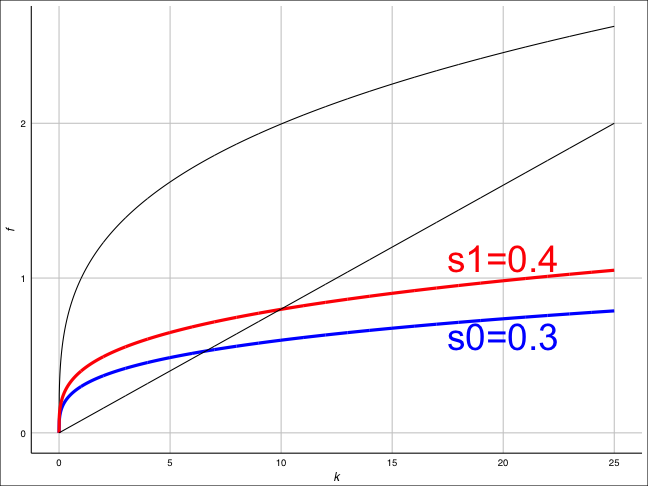### Comparative Statics

• $$k^*$$ is larger when $$s$$ gets larger.

• Policy that increases the saving rate increases GDP per capita: $\frac{Y}{L} = A f(k^*)$
• There is a level effect

• In the long run, there is no change: fixed at $$g$$.There is no growth effect.
• In the shorter run, there is some change.

### Comparative Dynamics

• Suppose that the economy is on the balanced growth path.
• i.e., $$k = k^*$$
• At time $$t_0$$, the economy experiences a sudden increase in $$s$$ (from s0 to s1).
• $$k$$ gradually moves toward the new $$k^*$$ because the new saving level is greater than the break-even level of investment.
• Investment per capita immediately jumps up to a point on the new (red) saving curve. (jump variable)
• Consumption per capita immediately falls because of the rise of investment. (jump variable)

### Simulation for k

k0 = (s0 / (g + n + delta))^(1 / (1 - alpha)) # steady state
t0 = 10  # Change of policy

solow_update = function(t, k){
if (t < t0){
return(k + dt * (s0 * f(k) - (delta + g + n) * k))
} else {
return(k + dt * (s1 * f(k) - (delta + g + n) * k))
}
}

dt = 0.01   # controls precision of approximation
t_max = 100   # simulation for t_max years
t = seq(from=0, to=t_max, by=dt)
simulation = as.data.frame(t)

simulation[1, "k"] = k0
for (i in 2:nrow(simulation)){
simulation[i, "k"] = solow_update(simulation[i-1, "t"],
simulation[i-1, "k"])
}


### Simulation for k

ggplot(simulation, aes(x=t, y=k)) + geom_line() + theme_gdocs()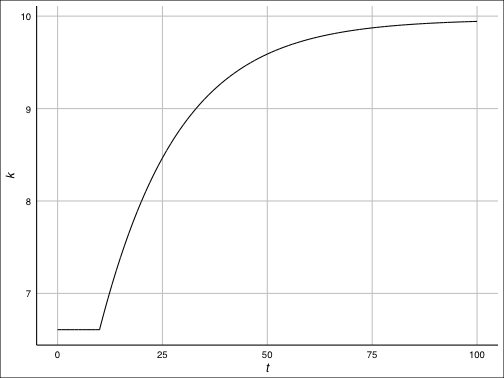At $$t = t_0$$, $$k$$ starts to increase and

it stops increasing when it attains the new steady state value.

### Simulation for K/L (log scale for y-axis)

Let $$A(0) = 1$$. Plot using a logarithmic scale for y-Axis.

simulation$KL = simulation$k * exp(g*simulation\$t)
ggplot(simulation, aes(x=t, y=KL)) + geom_line() + scale_y_log10() + theme_gdocs()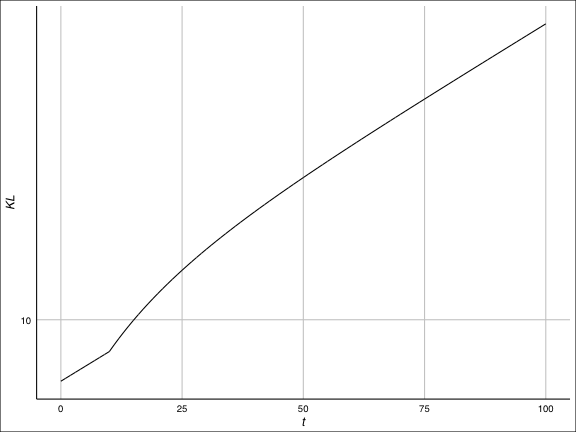### Simulation for C/L (log scale for y-axis)

Exercise: Reproduce the following graph.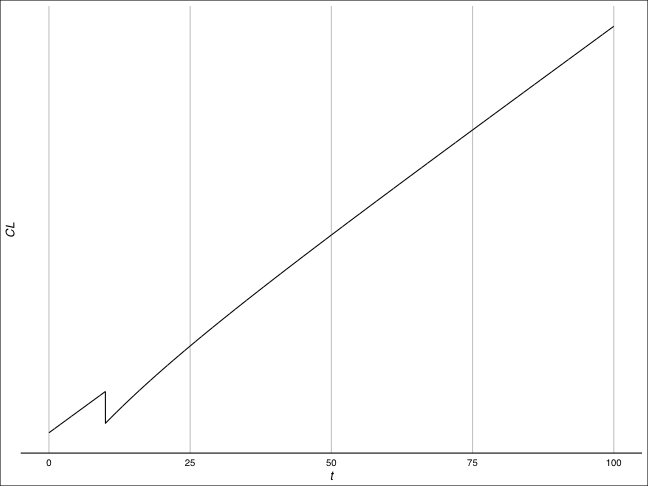### Transition Dynamics

Note that the growth rate, $$g_{K/L}$$ of $$K/L$$ satisfies

$g_{K/L}(t) = g + g_k(t),$

where $$g$$ is the exogenous growth rate of $$A$$, $$g_k$$ is the growth rate of $$k$$.

After an increase in saving rate, we get $$\dot k > 0$$ and thereby $$g_k(t) > 0$$.

On the transition path, the growth of per capita capital is faster than on the BGP.

It seems to be consistent with observations about NICs.

### Golden rule

• There is a certain value for $$s$$ that maximizes steady state consumption.
• Such saving rate is called Golden rule saving rate and denoted by $$s_G$$.
• For Cobb–Douglas production funtion $$f(k) = k^\alpha$$, $$s_G = \alpha$$. (Why?)

### Golden rule (cont'd)

Note that

\begin{aligned} c^* &= (1 - s) f(k^*) \\ &= f(k^*) - (\delta + g + n) k^* \end{aligned}

When $$c^*$$ is maximized, we should have (think of $$c^*$$ as a function of $$k^*$$)

$f'(k^*) = \delta + g + n$

Let $$k^*_G$$ be the unique stock level that satisfies the above equation. Golden rule capital stock.

### Simulation for C/AL

Exercise: Reproduce the following graph.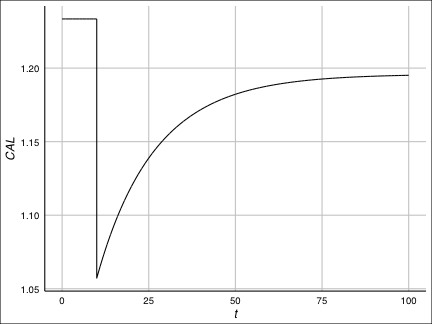Observe that the new steady state value, $$c^*$$, for $$c = C/(AL)$$ is smaller after the parameter change considered above.

• If $$s_0 < s_1 \le s_G$$, the parameter shift from $$s_0$$ to $$s_1$$ necessarily makes $$(C/AL)^*$$ larger after the shift.
• Confirm this fact with pen and paper, and with R.
• If $$s_G \le s_0 < s_1$$, $$(C/AL)^*$$ gets smaller.
• This is what we have observed.

### Dynamic Inefficiency

• Saving rate greater than the golden-rule level is unrealistic.
• If you lower the saving rate, you can increase consumption immediately and forever.
• There is dynamic inefficiency.

### Growth Accounting

$$Y = F(K, AL)$$ implies that

\begin{aligned} \dot Y &= \frac{\partial Y}{\partial K} \dot K + \frac{\partial Y}{\partial (AL)} \frac{d}{dt}(AL)\\ &= \frac{\partial Y}{\partial K} \dot K + \left[\frac{\partial Y}{\partial (AL)} A\right] \dot L + \left[\frac{\partial Y}{\partial (AL)} L\right] \dot A\\ &=: \frac{\partial Y}{\partial K} \dot K + \frac{\partial Y}{\partial L} \dot L + \left[\frac{\partial Y}{\partial (AL)} L\right] \dot A. \end{aligned}

We therefore have

$\frac{\dot Y}{Y} = \frac{\partial Y}{\partial K} \frac{K}{Y} \frac{\dot K}{K} + \frac{\partial Y}{\partial L} \frac{L}{Y} \frac{\dot L}{L} + \left[\frac{\partial Y}{\partial (AL)} \frac{AL}{Y} \right] g.$

### Growth Accounting (cont'd)

Define

\begin{aligned} \alpha_K (t) &:= \frac{K}{Y} \frac{\partial Y}{\partial K} & (\text{capital elasticity of output}) \\ \alpha_L (t) &:= \frac{L}{Y} \frac{\partial Y}{\partial L} & (\text{labor elasticity of output}) \end{aligned}

Veryfy for the Cobb–Douglas family that they are constant: $$\alpha_K = \alpha$$ and $$\alpha_L = 1-\alpha$$.

By Euler's theorem on CRS functions,

$\alpha_K (t) + \alpha_L (t) = 1$

### Growth Accounting (cont'd)

1% increase in capital input results in $$\alpha_K$$% increase in output.

$\alpha_K = \frac{K}{Y} \frac{\partial Y}{\partial K} \simeq \dfrac{ \dfrac{Y + \Delta Y}{Y} }{ \dfrac{K + \Delta K}{K} }$

### Growth Accounting (cont'd)

By employing this notation, the decomposition of $$\dot Y/Y$$ becomes

$\frac{\dot Y}{Y} = \alpha_K \frac{\dot K}{K} + \alpha_L \frac{\dot L}{L} + R,$

where

$R := \left[\frac{\partial Y}{\partial (AL)} \frac{AL}{Y} \right] g = \alpha_L g,$

called the Solow residual.

All terms other than $$R$$ can be obtained from data.

### Growth Accounting (cont'd)

Equivalently,

$g_{Y/L} = \alpha_K g_{K/L} + R = \alpha_K g_{K/L} + a_L g.$

In the steady state, $$\alpha_K$$ fraction of growth in output per worker is attributable to capital accumulation. The rest is due to the technological progress.

After extended to incorporate human capital accumulation, the Solow model fits fairly well with data. See Mankiw, Romer and Weil (1992, QJE).